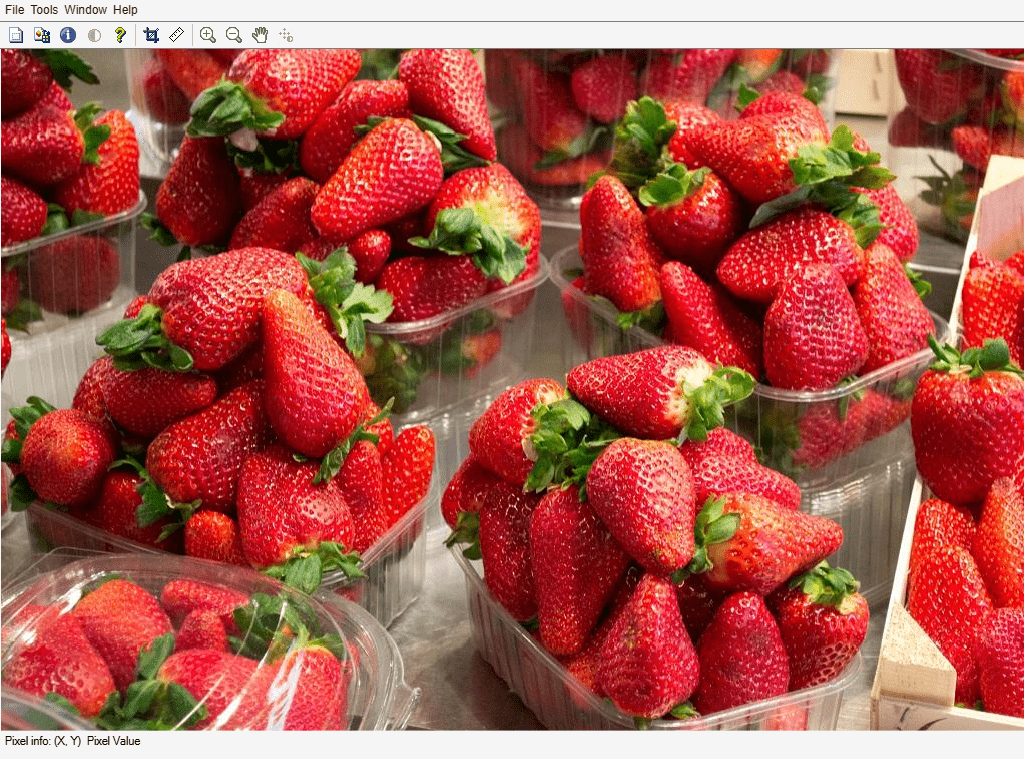# imgcf

Get current figure containing image

## Syntax

``fig = imgcf``

## Description

example

````fig = imgcf` returns the current figure that contains an image. The figure may be a regular figure window that contains at least one image or an Image Tool window.If none of the figures currently open contains an image, then `imgcf` creates a new figure.```

## Examples

collapse all

Open an image in an Image Tool window.

```I = imread('strawberries.jpg'); imtool(I)```Use the handle of a figure containing an Image Tool window to center the window on the screen.

```sz = get(groot,'ScreenSize'); pos = get(imgcf,'Position'); pos = [(sz(3)-pos(3))/2 (sz(4)-pos(4))/2 pos(3) pos(4)]; set(imgcf,'Position',pos)```## Output Arguments

collapse all

Current figure containing an image, returned as a `figure` object.

## Tips

• `imgcf` can be useful in getting the figure used by the Image Tool. You cannot retrieve the tool figure using `gcf`.